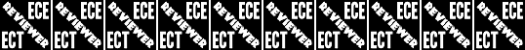# Ect001

20 Questions | Total Attempts: 36889SettingsPriority Level: High (ECE & ECT)

• 1.
How fast does a radio wave travel through free space?
• A.

At the speed of light

• B.

At the speed of sound

• C.

Its speed is inversely proportional to its wavelength

• D.

Its speed increases as the frequency increases

• 2.
How does the wavelength of a radio wave relate to its frequency?
• A.

The wavelength gets longer as the frequency increases

• B.

The wavelength gets shorter as the frequency increases

• C.

There is no relationship between wavelength and frequency

• D.

The wavelength depends on the bandwidth of the signal

• 3.
What property of radio waves is often used to identify the different frequency bands?
• A.

The approximate wavelength

• B.

The magnetic intensity of waves

• C.

The time it takes for waves to travel one mile

• D.

The voltage standing wave ratio of waves

• 4.
What are the frequency limits of the VHF spectrum?
• A.

30 to 300 kHz

• B.

30 to 300 MHz

• C.

300 to 3000 kHz

• D.

300 to 3000 MHz

• 5.
What are the frequency limits of the UHF spectrum?
• A.

30 to 300 kHz

• B.

30 to 300 MHz

• C.

300 to 3000 kHz

• D.

300 to 3000 MHz

• 6.
What frequency range is referred to as HF?
• A.

300 to 3000 MHz

• B.

30 to 300 MHz

• C.

3 to 30 MHz

• D.

300 to 3000 kHz

• 7.
How many volts are equal to one kilovolt?
• A.

One one-thousandth of a volt

• B.

One hundred volts

• C.

One thousand volts

• D.

One million volts

• 8.
Which of the following is equivalent to 500 milliwatts?
• A.

0.02 watts

• B.

0.5 watts

• C.

5 watts

• D.

50 watts

• 9.
If an ammeter calibrated in amperes is used to measure a 3000-milliampere current, what reading would it show?
• A.

0.003 amperes

• B.

0.3 amperes

• C.

3 amperes

• D.

3,000,000 amperes

• 10.
If a frequency readout calibrated in megahertz shows a reading of 3.525 MHz, what would it show if it were calibrated in kilohertz?
• A.

0.003525 kHz

• B.

35.25 kHz

• C.

3525 kHz

• D.

3,525,000 kHz

• 11.
What is the unit of frequency?
• A.

Hertz

• B.

Henry

• C.

• D.

Tesla

• 12.
What is the abbreviation that refers to radio frequency signals of all types?
• A.

AF

• B.

HF

• C.

RF

• D.

VHF

• 13.
What is the name for the distance a radio wave travels during one complete cycle?
• A.

Wave speed

• B.

Waveform

• C.

Wavelength

• D.

• 14.
What is the formula for converting frequency to wavelength in meters?
• A.

Wavelength in meters equals frequency in hertz multiplied by 300

• B.

Wavelength in meters equals frequency in hertz divided by 300

• C.

Wavelength in meters equals frequency in megahertz divided by 300

• D.

Wavelength in meters equals 300 divided by frequency in megahertz

• 15.
What is the approximate velocity of a radio wave as it travels through free space?
• A.

3000 kilometers per second

• B.

300,000,000 meters per second

• C.

300,000 miles per hour

• D.

186,000 miles per hour

• 16.
How many milliamperes is 1.5 amperes?
• A.

15 milliamperes

• B.

150 milliamperes

• C.

1,500 milliamperes

• D.

15,000 milliamperes

• 17.
What is another way to specify a radio signal frequency of 1,500,000 hertz?
• A.

1500 kHz

• B.

1500 MHz

• C.

15 GHz

• D.

150 kHz

• 18.
How many volts are equal to one microvolt?
• A.

One one-millionth of a volt

• B.

One million volts

• C.

One thousand kilovolts

• D.

One one-thousandth of a volt

• 19.
• A.

• B.

• C.

• D.

• 20.
What determines the amount of deviation of an FM (as opposed to PM) signal?
• A.

Both the frequency and amplitude of the modulating signal

• B.

The frequency of the modulating signal

• C.

The amplitude of the modulating signal

• D.

The relative phase of the modulating signal and the carrier

Related TopicsBack to top